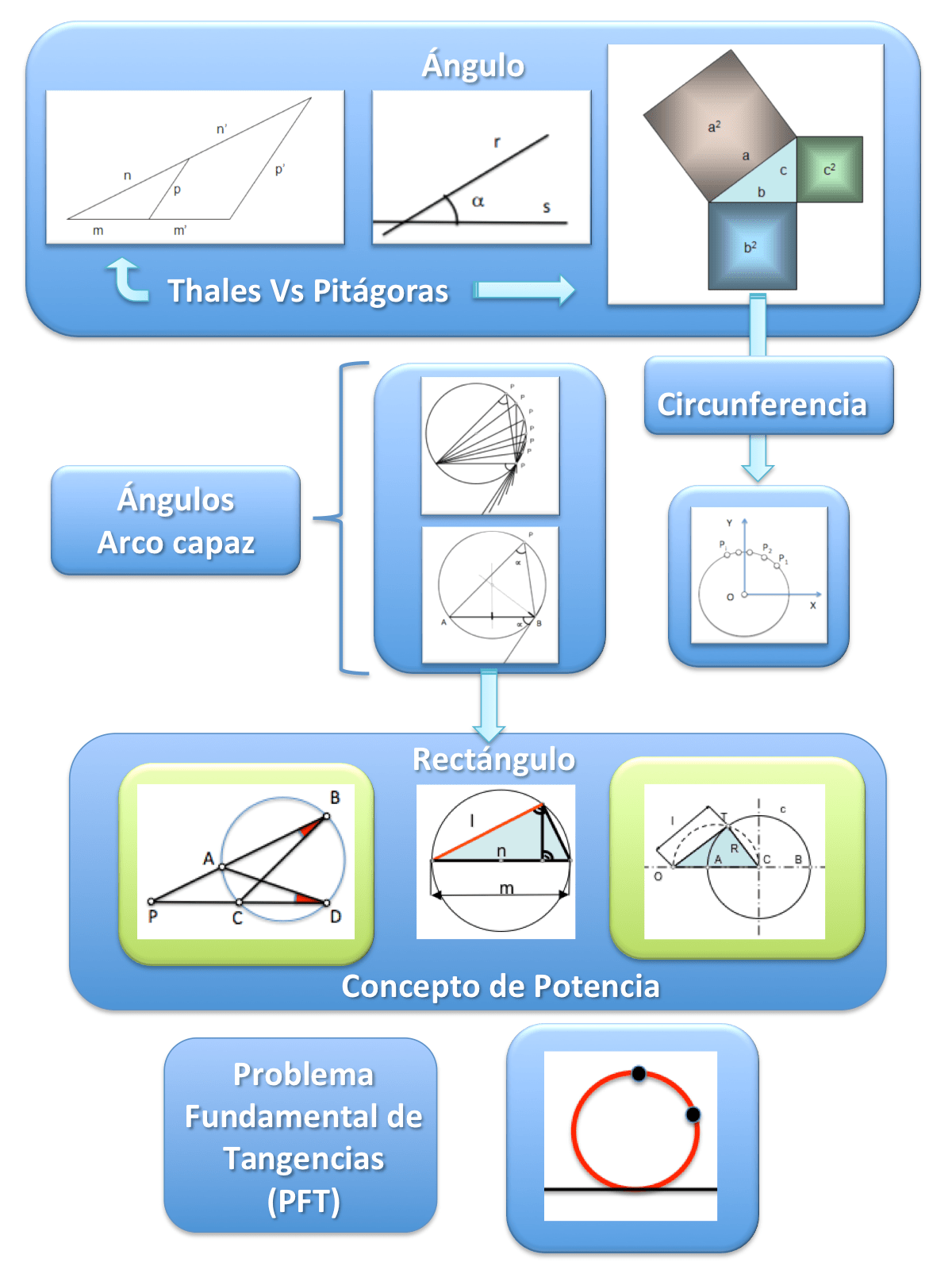# Learning Path Metric GeometryIn addressing the study of a science we can follow different paths that lead to learning. Chaining concepts linked to each other allow us to generate a mental representation of abstract patterns, facilitating their assimilation and subsequent application in problem solving.

The geometry is not much different in this respect to other disciplines yet, at initial levels of introduction in high school it has been “described” as a set of “Mechanistic nature paths” that solve the problems without adequate justification. Far from this interpretation, some geometry treaties establish training pathways that simplify learning of this science.

The geometry (Latin geometry, which comes from the Greek language γεωμετρία, geo landmetria measure), is a branch of mathematics that deals with the study of the properties of geometric figures in the plane or space, like: points, straight, planes, polytopes (including parallel, perpendicular, curves, surfaces, polygons,polyhedra, etc.).(W)

In these pages two images are proposed summarizing a possible strategy or sequence gradually incorporating the basics of this branch of science in the education of our students.

At first the basics educational level would be set on which further developments will be supported:

• Theorem of Thales
• Pythagorean Theorem
• Arco able
• Power Concepts
• Fundamental Problem TangenciesTras la incorporación de los conceptos básicos anteriores podemos avanzar en el estudio vertical de detalle de los conceptos básicos aprendidos. So, el concepto elemental de “power” nos permitirá acometer el “fundamental problem of tangents” en cualquiera de sus variantes, y la incorporación del concepto de “Beam circumferences” facilitará una generalización más amplia del mismo.Esta secuencia puede completarse posteriormente con el estudio clásico de los problemas de tangencias y el estudio métrico de las cónicas.

Metric geometry